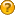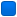###Author Topic: Random() and Randomize implementation  (Read 3044 times)

#### joselitosn

• Guest##### Random() and Randomize implementation
« on: April 01, 2007, 07:11:18 am »
I found a macro on the web to use random() and randomize(), but it does'nt seem to work.

It's likely to be a problem with the pseudo-random number generator.

Quote
#include <stdio.h>
#include <stdlib.h>
#include <time.h>

/****** snippet from comp.lang.c ******/
#ifndef __TURBOC__
#define random( num ) ( int ) ((( rand()) * ( long ) ( num )) / ((( long ) RAND_MAX ) + 1 ))
#define randomize() srand(( unsigned ) time( NULL ) | 1 )
#endif

int main( void )
{
unsigned long long int x, y, z; // 64 bit, right?

randomize();

x = RAND_MAX;
printf("That's RAND_MAX : %llu", x );
getchar();

printf("This number should be random in the interval from 0 to 52 : %d", random( 52 ));
getchar();

/****** now i do the same as the random macro ******/

y = rand() * 52; // 52 is the max number i want
x += 1;
z = (long long int) ((long double)y / x); // convert double for division and convert back? Error?

printf("The result of %llu divided by %llu results in %llu ?", y, x, z );
getchar();

return( 0 );
}

Tested with Ch Interpreter and lcc-win32, worked fine in both. The visible difference is the RAND_MAX which is 32767.

Also is there any snippet for the sound() and delay() functions?
« Last Edit: April 01, 2007, 08:13:58 am by joselitosn »

####frankie

• Global Moderator
• Member
••• Posts: 1769##### Re: Random() and Randomize implementation
« Reply #1 on: April 01, 2007, 12:29:31 pm »
Yuor problem is due to the fact that other compilers returns a short as result (16bits integer max positive value=32768). PellesC returns a real 32 bits int that is equivalent to long (32 bits).
To let work the code just change the casting to long:
Code: [Select]
`/****** snippet from comp.lang.c ******/#ifndef __TURBOC__   #define random( num ) ( int ) ((( (long long)rand()) * ( long long ) ( num )) / ((( long long ) RAND_MAX ) + 1 ))   #define randomize() srand(( unsigned ) time( NULL ) | 1 )#endifint main( void ){   unsigned long long int x, y, z; // 64 bit, right? int i;      randomize();   x = RAND_MAX;   printf("That's RAND_MAX : %llu (0x%x)", x, x );   getchar();    for (i=0; i<52; i++)    printf("This number should be random in the interval from 0 to 52 : %d\n", random( 52 ));   getchar();/****** now i do the same as the random macro ******/   y = (long long)rand() * 52; // 52 is the max number i want   x += 1;   z = (long long int) ((long long)y / x); // convert double for division and convert back? Error?   printf("The result of %llu divided by %llu results in %llu ?", y, x, z );   getchar();   return( 0 );}`Or if you prefer reduce the rand output to 16 bits. The last will let increase the frequency for lower values in requested interval.

#### joselitosn

• Guest##### Re: Random() and Randomize implementation
« Reply #2 on: April 01, 2007, 07:43:25 pm »
I thought it should be an implicit type conversion. But now i understood. Thanks!

EDIT: If i got it right "unsigned int" should have the same size as "unsigned long int" in Pelles C compiler, so i should use "unsigned long int" for compatibility (unless i can change the RAND_MAX or the max number rand() can give).

If i choose to use "unsigned long int", "unsigned long long int" need to be used only for "rand() * num" as this results into a larger number.

Should this be the optimal solution for compatibility with all compilers?
Quote
#ifndef __TURBOC__
#define randomize() srand((unsigned int)time((time_t *)NULL))
#define random(num) (unsigned long int)((((unsigned long long int)rand())*((unsigned long int)(num)))/(((unsigned long int)RAND_MAX)+1))
#endif

Later i'm going to find a PRNG library, but now i can only use that.
« Last Edit: April 02, 2007, 03:09:25 am by joselitosn »